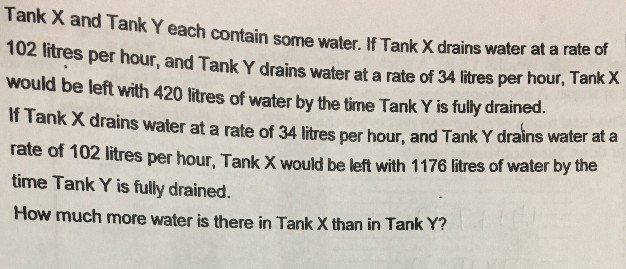# QuestionPls help. Thanks!

2 Answers

# Answer

Case 1
X : Y => 102 : 34

Case 2
X : Y => 34 : 102

both cases, Tank Y drained the same volume till the end.

Times 3 to Case 1 to make Tank Y similar to Case 2. So they share common units ‘u’
Case 1
X : Y => 306 : 102

Water in Tank X can be represented from the two cases
X = 306u + 420 from case 1
X = 34u + 1176 from case 2

306u + 420 = 34u + 1176
272u = 756
u = 756/272

Tank X = 306u + 420 = 1270.5 L
Tank Y = 102u = 283.5 L
Difference = 1270.5 – 283.5 = 987 L ##

1 Reply 0 Likes ✔Accepted Answer

1u in this case represents hours isn’t it? that would result in fractions of a second. i thought primary school questions are supposed to “make sense”? 🙁

0 Replies 0 Likes

Scenario 1 : If Tank X drains water at a rate of 102 litres per hour and Tank Y drains water at a rate of 34 litres per hour, Tank X would be left with 420 litres of water by the time Tank Y is fully drained.
Tank X : 102, 204, 306, … , 420 left
Tank Y : 34, 68, 102, … 0

Scenario 2 : If Tank X drains water at a rate of 34 litres per hour and Tank Y drains water at a rate of 102 litres per hour, Tank X would be left with 1176 litres of water by the time Tank Y is fully drained.
Tank X : 34, 68, 102,, … , 1176 left
Tank Y : 102, 204, 306, … 0

Method 1 :
1176 – 420 = 756
306 – 34 = 272
(756/272) x 34 + 1176 = 1270.5
(756/272) x 102 = 283.5
1270.5 – 283.5 = 987

Method 2 :
34 x number of hours in Scenario 1 = 102 x number of hours in Scenario 2 (Tank Y)
number of hours in Scenario 1 = 3 x number of hours in Scenario 2 (Tank Y)
102 x number of hours in Scenario 1 + 420 = 34 x number of hours in Scenario 2 + 1176
306 x number of hours in Scenario 2 + 420 = 34 x number of hours in Scenario 2 + 1176

number of hours in Scenario 2 = (1176 – 420)/(306 – 34) = 756/272
(756/272) x 34 + 1176 = 1270.5 or (756/272) x 3 x 102 + 420 = 1270.5
(756/272) x 102 = 283.5 or (756/272) x 3 x 34 = 283.5
1270.5 – 283.5 = 987

Ans : 987 litres more.

0 Replies 0 Likes